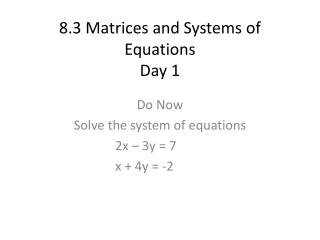DownloadDownload Presentation8.3 Matrices and Systems of Equations Day 1

# 8.3 Matrices and Systems of Equations Day 1

Download Presentation## 8.3 Matrices and Systems of Equations Day 1

- - - - - - - - - - - - - - - - - - - - - - - - - - - E N D - - - - - - - - - - - - - - - - - - - - - - - - - - -
##### Presentation Transcript

1. 8.3 Matrices and Systems of EquationsDay 1 Do Now Solve the system of equations 2x – 3y = 7 x + 4y = -2

2. HW Review: 8.2 #1-23

3. Matrices and Systems • We can use an augmented matrix to represent systems of equations • Ex: 2x – 3y = 7 x + 4y = -2

4. Matrices • The rows of a matrix are horizontal • The columns are vertical • A matrix with m rows and n columns is said to be of order m x n • The numbers in a matrix are called entries • The main diagonal starts from the top left and travels down and to the right

5. Gaussian Elimination with Matrices • If we write a system of equations as an augmented matrix, we can use row-equivalent operations to write a matrix with a main diagonal of 1’s

6. Row-Equivalent Operations • In a matrix, you are allowed to: • Interchange any two rows • Multiply each entry in a row by the same nonzero constant • Add a nonzero multiple of one row to another row

7. Ex • Solve the system of equations 2x – y + 4z = -3 x – 2y – 10z = -6 3x + 4z = 7

8. Ex • Solve the systems of equations using matrices x – y+ 2z = 0 x – 2y + 3z = -1 2x – 2y + z = -3

9. Closure • Solve the system using matrices 4x – 8y = 12 -x + 2y = -3 • HW: p.710 #1-13 odds, 15-39 EOO

10. 8.3 Matrices and SystemsDay 2 • Do Now • Solve the system using matrices 3x + 2y + 2z = 3 x + 2y – z = 5 2x – 4y + z = 0

11. HW Review: p.710 #1-13, 15-39 EOO

12. Row-Echelon Form • A matrix is in row-echelon form, then: • 1) The first nonzero element in each row is a 1 • 2) For any two successive nonzero rows, the leading 1 in the lower row is farther to the right than the leading 1 in the higher row • 3) All the rows consisting entirely of 0’s are at the bottom of the matrix • Note: a matrix is said to be in reduced row-echelon form if: • Each column that contains a leading 1 has 0’s everywhere else (except the last column of an augmented matrix)

13. Ex • Ex2 on p.707 in book

14. Gauss-Jordan Elimination • In this method, we want to write each system of equations as an augmented matrix in reduced row-echelon form

15. Ex • Solve the system of equations 3x – 4y – z = 6 2x – y + z = -1 4x – 7y – 3z = 13

16. Closure • Solve the system of equations 2x – 3y + 2z = 3 x + 4y – z = 9 3x + y – 5z = 5 • HW: p.710 #15-39 AOO

17. 8.3 Matrices and SystemsDay 3 • Do Now • Solve the system of equations x + y – 3z = 4 4x + 5y + z = 1 2x + 3y + 7z = -7

18. HW Review p.710 #15-39 AOO

19. Closure • Would you rather use matrices or algebraic methods to solve a system of linear equations in 2/3 variables? Why? • 8.1-8.3 Quiz Friday

20. 8.1-8.3 Review • Do Now • Solve the system of equations by any means x – y + z = 3 2x + y – 3z = 5 4x + y – z = 11

21. 8.1-8.3 Review • 8.1 Solving Linear Systems in 2 variables • Graphing, Substitution, Elimination • 8.2 Solving Linear Systems in 3 Variables • Substitution or Elimination • 8.3 Using Matrices to Solve Systems • Elimination using Row-Equivalent Operations

22. Closure • Solve this system of equations using as many methods as possible 2x + y = 1 3x + 2y = -2 8.1-8.3 Quiz Tomorrow